# How to count unique values in Excel

In this tutorial, you will learn how to count unique values in Excel with formulas, and how to get an automatic count of distinct values in a pivot table. We will also discuss a number of formula examples for counting unique names, texts, numbers, cased-sensitive unique values, and more.

When working with a large dataset in Excel, you may often need to know how many duplicate and unique values are there. And sometimes, you may want to count only the distinct (different) values.

If you have been visiting this blog on a regular basic, you already know the Excel formula to count duplicates. And today, we are going to explore different ways to count unique values in Excel. But for the sake of clarity, let's define the terms first.

• Unique values - these are the values that appear in the list only once.
• Distinct values - these are all different values in the list, i.e. unique values plus 1st occurrences of duplicate values.

The following screenshot demonstrates the difference: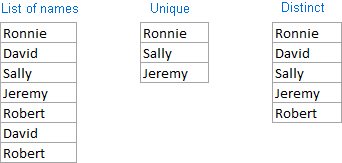And now, let's see how you can count unique and distinct values in Excel using formulas and PivotTable features.

## How to count unique values in Excel

Here's a common task that all Excel users have to perform once in a while. You have a list of data and you need to find out the number of unique values in that list. How do you do that? Easier than you may think :) Below you will find a few formulas to count unique values of different types.

### Count unique values in a column

Supposing you have a column of names in your Excel worksheet, and you need to count unique names in that column. The solution is to use the SUM function in combination with IF and COUNTIF:

=SUM(IF(COUNTIF(range, range)=1,1,0))

Note. This is an array formula, so be sure to press Ctrl + Shift + Enter to complete it. Once you do this, Excel will automatically enclose the formula in {curly braces} like in the screenshot below. In no case should you type the curly braces manually, that won't work.

In this example, we are counting unique names in range A2:A10, so our formula takes the following shape:

`=SUM(IF(COUNTIF(A2:A10,A2:A10)=1,1,0))`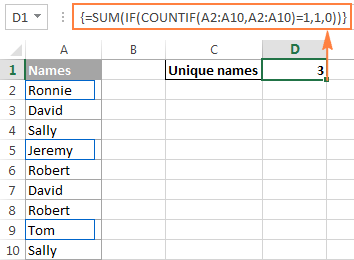Further on in this tutorial, we are going to discuss a handful of other formulas to count unique values of different types. And because all those formulas are variations of the basic Excel unique values formula, it makes sense to break down the above formula, so you can fully understand how it works and tweak it for your data. If someone is not interested in technicalities, you can skip right to the next formula example.

#### How the Excel count unique values formula works

As you see, 3 different functions are used in our unique values formula - SUM, IF and COUNTIF. Looking from the inside out, here's what each function does:

• The COUNTIF function counts how many times each individual value appears in the specified range.

In this example, `COUNTIF(A2:A10,A2:A10)` returns the array `{1;2;2;1;2;2;2;1;2}`.

• The IF function evaluates each value in the array returned by COUNTIF, keeps all 1's (unique values), and replaces all other values with zeros.

So, the function `IF(COUNTIF(A2:A10,A2:A10)=1,1,0)` becomes `IF(1;2;2;1;2;2;2;1;2) = 1,1,0,` which turns into the array `{1;0;0;1;0;0;0;1;0}` where 1 is a unique value and 0 is a duplicate value.

• Finally, the SUM function adds up the values in the array returned by IF and outputs the total number of unique values, which is exactly what we wanted.

Tip. To see what a specific part of your Excel unique values formula evaluates to, select that part in the formula bar and press the F9 key.

### Count unique text values in Excel

If your Excel list contains both numerical and text values, and you want to count only unique text values, add the ISTEXT function to the array formula discussed above:

`=SUM(IF(ISTEXT(A2:A10)*COUNTIF(A2:A10,A2:A10)=1,1,0))`

As you know, the Excel ISTEXT function returns TRUE if an evaluated value is text, FALSE otherwise. Since the asterisk (*) works as the AND operator in array formulas, the IF function returns 1 only if a value is both text and unique, 0 otherwise. And after the SUM function adds up all 1's, you will get a count of unique text values in the specified range.

Don't forget to press Ctrl + Shift + Enter to correctly enter the array formula, and you will get a result similar to this: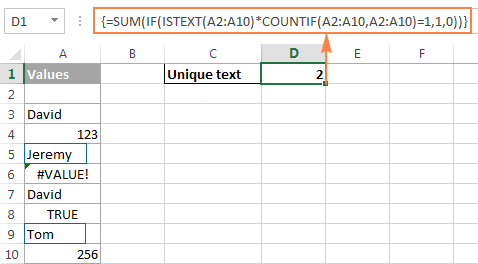As you can see in the screenshot above, the formula returns the total number of unique text values, excluding blank cells, numbers, logical values of TRUE and FALSE, and errors.

### Count unique numeric values in Excel

To count unique numbers in a list of data, utilize an array formula like we've just used for counting unique text values, with the only difference that you embed ISNUMBER instead of ISTEXT in your unique values formula:

`=SUM(IF(ISNUMBER(A2:A10)*COUNTIF(A2:A10,A2:A10)=1,1,0))`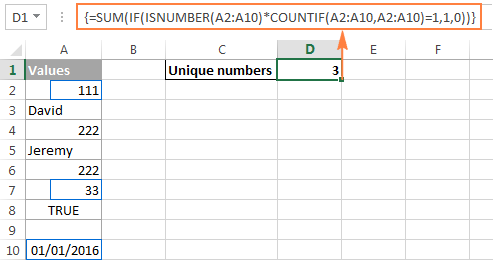Note. Since Microsoft Excel stores dates and times as serial numbers, they are also counted.

### Count case-sensitive unique values in Excel

If your table contains case-sensitive data, the easiest way to count unique values would be creating a helper column with the following array formula to identify duplicate and unique items:

`=IF(SUM((--EXACT(\$A\$2:\$A\$10,A2)))=1,"Unique","Dupe")`

And then, use a simple COUNTIF function to count unique values:

`=COUNTIF(B2:B10, "unique")`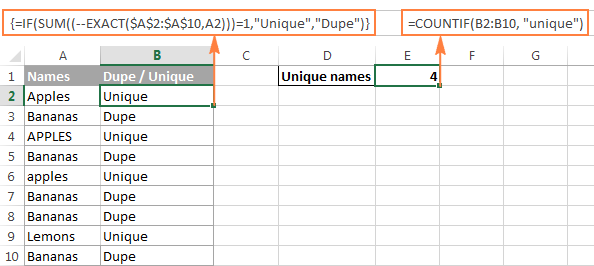## Count distinct values in Excel (unique and 1st duplicate occurrences)

To get a count of distinct values in a list, use the following formula:

=SUM(1/COUNTIF(range, range))

Remember, it's an array formula, and therefore you should press the Ctrl + Shift + Enter shortcut instead of the usual Enter keystroke.

Alternatively, you can use the SUMPRODUCT function and complete the formula in the usual way by pressing the Enter key:

=SUMPRODUCT(1/COUNTIF(range, range))

For example, to count the distinct values in range A2:A10, you can go with either:

`=SUM(1/COUNTIF(A2:A10,A2:A10))`

Or

`=SUMPRODUCT(1/COUNTIF(A2:A10,A2:A10))`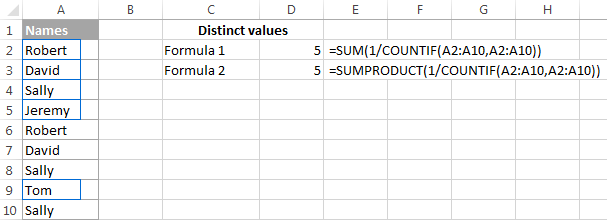#### How the Excel distinct formula works

As you already know, we use the COUNTIF function to find out how many times each individual value appears in the specified range. In the above example, the result of the COUNTIF function is the following array: `{2;2;3;1;2;2;3;1;3}`.

After that, a number of division operations are performed, where each value of the array is used as a divisor with 1 as the dividend. This turns all duplicates values into fractional numbers corresponding to the number of duplicate occurrences. For example, if a value appears 2 times in the list, it generates 2 items in the array with a value of 0.5 (1/2=0.5). And if a value appears 3 times, it produces 3 items in the array with a value of 0.3(3). In our example, the result of `1/COUNTIF(A2:A10,A2:A10))` is the array `{0.5;0.5;0.3(3);1;0.5;0.5;0.3(3);1;0.3(3)}`.

Doesn't make much sense so far? That's because we haven't applied the SUM / SUMPRODUCT function yet. When one of these functions adds up the values in the array, the sum of all fractional numbers for each individual item always yields 1, no matter how many occurrences of that item exist in the list. And because all unique values appear in the array as 1's (1/1=1), the final result returned by the formula is the total number of all different values in the list.

### Formulas to count distinct values of different types

As is the case with counting unique values in Excel, you can use variations of the basic Excel count distinct formula to handle specific value types such as numbers, text, and case-sensitive values.

Please remember that all of the below formulas are array formulas and require pressing Ctrl + Shift + Enter.

#### Count distinct values ignoring empty cells

If a column where you want to count distinct values might contain blank cells, you should add an IF function that will check the specified range for blanks (the basic Excel distinct formula discussed above would return the #DIV/0 error in this case):

=SUM(IF(range<>"",1/COUNTIF(range, range), 0))

For example, to count distinct values in range A2:A10, use the following array formula:

`=SUM(IF(A2:A10<>"",1/COUNTIF(A2:A10, A2:A10), 0))`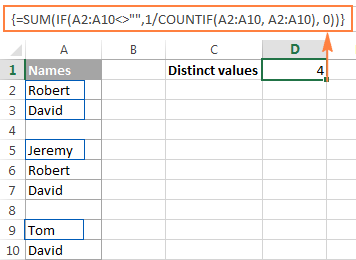#### Formula to count distinct text values

To count distinct text values in a column, we'll be using the same approach that we've just used to exclude empty cells.

As you can easily guess, we will simply embed the ISTEXT function into our Excel count distinct formula:

=SUM(IF(ISTEXT(range),1/COUNTIF(range, range),""))

And here's a real-life formula example:

`=SUM(IF(ISTEXT(A2:A10),1/COUNTIF(A2:A10, A2:A10),""))`

#### Formula to count distinct numbers

To count distinct numeric values (numbers, dates and times), use the ISNUMBER function:

=SUM(IF(ISNUMBER(range),1/COUNTIF(range, range),""))

For example, to count all different numbers in range A2:A10, use the following formula:

`=SUM(IF(ISNUMBER(A2:A10),1/COUNTIF(A2:A10, A2:A10),""))`

#### Count case-sensitive distinct values in Excel

Similarly to counting case-sensitive unique values, the easiest way to count case-sensitive distinct values is to add a helper column with the array formula that identifies unique values including first duplicate occurrences. The formula is basically the same as the one we used to count case-sensitive unique values, with one small change in a cell reference that makes a great difference:

`=IF(SUM((--EXACT(\$A\$2:\$A2,\$A2)))=1,"Distinct","")`

As you remember, all array formulas in Excel require pressing Ctrl + Shift + Enter.

After the above formula is finished, you can count "distinct" values with a usual COUNTIF formula like this:

`=COUNTIF(B2:B10, "distinct")`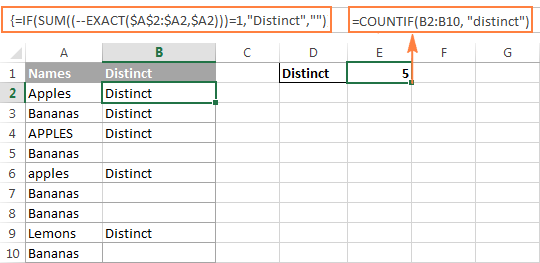If there is no way you can add a helper column to your worksheet, you can use the following complex array formula to count case-sensitive distinct values without creating an additional column:

`=SUM(IFERROR(1/IF(\$A\$2:\$A\$10<>"", FREQUENCY(IF(EXACT(\$A\$2:\$A\$10, TRANSPOSE(\$A\$2:\$A\$10)), MATCH(ROW(\$A\$2:\$A\$10), ROW(\$A\$2:\$A\$10)), ""), MATCH(ROW(\$A\$2:\$A\$10), ROW(\$A\$2:\$A\$10))), 0), 0))`

## Count unique and distinct rows in Excel

Counting unique / distinct rows in Excel is akin to counting unique and distinct values, with the only difference that you use the COUNTIFS function instead of COUNTIF, which lets you specify several columns to check for unique values.

For example, to count unique or distinct names based on the values in columns A (First Name) and B (Last Name), use one of the following formulas:

Formula to count unique rows:

`=SUM(IF(COUNTIFS(A2:A10,A2:A10, B2:B10,B2:B10)=1,1,0))`

Formula to count distinct rows:

`=SUM(1/COUNTIFS(A2:A10,A2:A10,B2:B10,B2:B10))`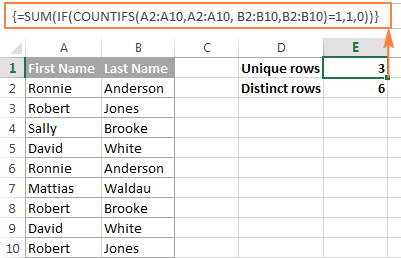Naturally, you are not limited to counting unique rows based only on two columns, the Excel COUNTIFS function can process up to 127 range/criteria pairs.

## Count distinct values in Excel using a PivotTable

The latest versions of Excel 2013 and Excel 2016 have a special feature that allows counting distinct values automatically in a pivot table. The following screenshot gives an idea of how the Excel Distinct Count looks like: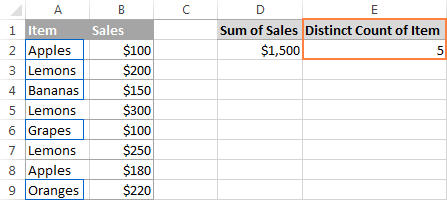To create a pivot table with the distinct count for a certain column, perform the following steps.

1. Select the data to be included in a pivot table, switch to the Insert tab, Tables group, and click the PivotTable button.
2. In the Create PivotTable dialog, choose whether to place your pivot table in a new or existing worksheet, and be sure to select the Add this data to the Data Model checkbox.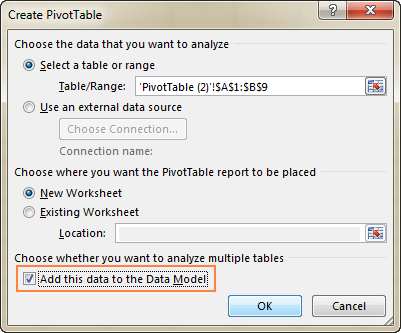3. When your pivot table opens, arrange the Rows, Columns and Values areas the way you want. If you don't have much experience with Excel pivot tables, the following detailed guidelines may prove helpful: Creating a PivotTable in Excel.
4. Move the field whose distinct count you want to calculate (Item field in this example) to the Values area, click on it, and select Field Value Settings… from the drop-down menu: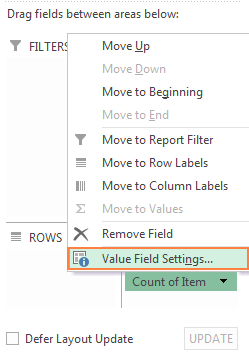5. The Value Field Settings dialog window will open, you scroll down to Distinct Count, which is the very last option in the list, select it and click OK.

You can also give a custom name to your Distinct Count if you want to.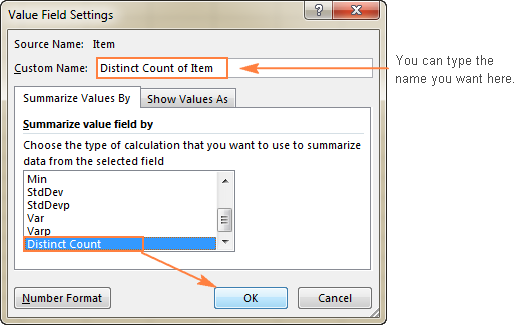Done! The newly created pivot table will display the distinct count like shown in the very first screenshot in this section.

Tip. After updating your source data, remember to update the PivotTable to bring the distinct count up to date. To refresh a pivot table, just click the Refresh button on the Analyze tab, in the Data group.

This is how you count distinct and unique values in Excel. If someone wants to have a closer look at the formulas discussed in this tutorial, you are welcome to download the sample Excel Count Unique workbook.

I thank you for reading and hope to see you again next week. In the next article, we are going to discuss various ways to find, filter, extract and highlights unique values in Excel. Please stay tuned!

1. If I have a list of numbers (a column that represent the number of days receivable outstanding - column Days Outstanding), how do I have excel count the numbers in a specified range (Age Ranges) and enter the results in (Expected results)). For example

Days Outstanding Age Ranges Expected Results
35 0-20 1
53 21-40 1
60 41-60 2
5 61-80 1
75 81-100 1
100

Thanks much

2. Column A Column B Column C
Student No. Student No BA No of occurrences
79700001 79700001 4
79700001 79700002 5
79700001 79700003 4
79700001 79700004 8
79700002 79700005 7
79700002 79700006 7
79700002 79700007
79700002 79700008
79700002 79700009

Which formula to use to count the of occurrences per student. The total to in Column C, as per example (manual)

3. Hi

I want to use sumıf functions but my number is 20 character that's why my formula dosent work. These are the unique numbers but sumıf functions seems whole number are same

88662511009190000314 = SUMIF(Z:Z;AE3;AA:AA) = 21 :(

88662511009190000314
88662511009190000315
88662511009190000316
88662511009190000317
88662511009190000318
88662511009190000319
88662511009190000320
88662511009190000321
88662511009190000322
88662511009190000323
88662511009190000324
88662511009190000325
88662511009190000326
88662511009190000327
88662511009190000328
88662511009190000329
88662511009190000330
88662511009190000331
88662511009190000332
88662511009190000333
88662511009190000334

• Hello! Unfortunately, Excel does not work with numbers longer than 15 characters.

4. 15039819 469072
15039819 418462
15039844 616305
15039844 723803
15039844 723803

i expect result

15039819 469072 2
15039819 418462 2
15039844 616305 2
15039844 723803 2
15039844 723803 2

i have to count how many unique value in column B have for every value of column 1.
Thanks

• Hi! Look for the example formulas here: Count unique values with criteria.

=IFERROR(ROWS(UNIQUE(FILTER(\$B\$1:\$B\$5,\$A\$1:\$A\$5=A1))), 0)

5. How do I do a unique count of products by supplier.

I have a distinct list of suppliers, and then a data summary of products used in a seperate table.

I've tried =if(table2[supplier]=a3,dcount(table2[product description],0) but it's not working??

6. Hi, how do I count the number of unique items in a column that fall between 2 dates. For example, how do I count each invoice number that falls between 1/1/22-2/1/22 . I was able to figure out how to count the number of invoices that fall within that date, but now I need to find the number of invoices that fall within that period.

7. I feel stupid. I put this formula in, and replace the range of 50 numbers with many distinct values, and the result is 1. I don't know what I'm doing wrong.

=SUM(IF(ISTEXT(C2:C50),1/COUNTIF(C2:C50, C2:C50),""))

• This formula counts the number of unique text values in the range.

8. Hi,
I have a column where each cell has multiple values separated by commas. Is there a way to list out each distinct value in each cell and display a count next to it? For example, in cell A1 I have "WTG, WTG, WTH, WTG, WTH". In B1, I want to show "WTG (3), WTH (2)".

Thank you!

9. Is it possible to combine a countif/ sumif formula? I have a list of different names in column 'A' and another list in column 'H'.

Example of what I need:

If the name in column 'A' is Andrew, sum the distinct values in column 'H'.

10. Hi All,

I have an excel where I need an formula to determine the count of projects from column A (there are duplicate values in this column)
This has to be combinated with the name of the Project Manager from column F.

Data looks like this

PS230000 - John Doe
PS230000 - John Doe
PS230000 - John Doe
PS230001 - Jane Doe
PS230001 - Jane Doe
PS230002 - John Doe

For the count of the projects I used =SUMPRODUCT((B5:B130"")/COUNTIF(B5:B130;B5:B130&"")) but I dont know how to add this to the name of the Project Manager so that I know how many projects John has and how many projects Jane has.

• Hello!
Try this formula:

=IFERROR(ROWS(UNIQUE(FILTER(A2:A10,B2:B10="John Doe"))), 0)

• Hi,

Thank you very much! unfortunately this won't work.
I forgot to mention that the Excel I have available is 2019 so the FILTER function does not exist.

11. Hello! I am struggling to find the right formula or table to give me the calculation I want. I'm hoping you can help! Column A has the dates, column E has a list of school district names - same school district name is used multiple times, and column I has the number of tests that have been started. I need the count of how many distinct school districts have started (I>0) as of a specific date. For example, the data looks a bit like this:
Date District Name Total Student Started
3/20/2023 K-12 Schools #1 0
3/20/2023 K-12 Schools #1 13
3/20/2023 K-12 Schools #2 33
3/20/2023 K-12 Schools #2 2
3/20/2023 K-12 Schools #3 2
3/20/2023 K-12 Schools #3 34

• Hello!

=IFERROR(ROWS(UNIQUE(FILTER(E2:E10,A2:A10=A1))), 0)

Подробные инструкции читайте в этой статье: How to count unique values with criteria Hope this is what you need.

• Oh my gosh, yes! That's exactly what I wanted!

12. Greetings!
I have a column in which numbers appear in an increasing sequence, example:
(Y20:Y30) - 1,6,9,12,13,18,24,31,31,36,36, ...
I need a formula that will show in the second column in the corresponding row the number of occurrences of numbers by increasing value > of 20, for example:
(Z20:Z30) - 0,0,0,0,0,0,1,2,0,1,0, ...
This example should display (Z20:Z30) for the numbers that appeared in (Y20:Y30). As can be seen in the example, the problem arises when the same values appear, (31,31,/36,36). When the same number appears to me, perhaps several times, then "0" or "" an empty cell should be displayed, and for further occurrences of increasing values, counting in order should be continued.
Example : - 0,0,0,0,0,0,1,2,0,1,0, ... 3,4,5,6, ... I tried a lot of formulas and of course I didn't succeed.. . i tried : =IF(Y20:Y30>29,Y20:Y30,"")
=COUNTIFS(Y19:Y20,">30") / =COUNTIFS(Y19:Y21,">30") / =COUNTIFS(Y19:Y22,">30")/=COUNTIFS(Y19:Y23,">30" )/Y24, ...Y30.
=IF(AB20>AB19,AB20,"0") / =IF(AB21>AB20,AB21,"0") /
=IF(AB22>AB21,AB22,"0") / ...
=IF(Y27>Y26,Y27,"0") / =IF(Y28>Y27,Y28,"0") / =IF(Y29>Y28,Y29,"0") Help Goran

• Hi!
If I understand your task correctly, the following formula should work for you:

=(COUNTIFS(\$Y\$20:\$Y20,Y20)=1)*(Y20>20)*(COUNTIF(\$Z\$19:Z19,">0")+1)

• I am very grateful to you! Works !

13. Hi I have a tables with dates in column a, projects in column B, and employee names in column c. The employee names repeat on the table and so do the project.

I have a another table with the project names removed with duplicates. I need help with a formula that would count how many employees worked on that job, leaving out duplicates, based on the project name in the column beside it. Any suggestions?

• Hello!
To count the number of employees working on the project, try this formula

=IFERROR(ROWS(UNIQUE(FILTER(C1:C10,B1:B10=F1))), 0)
F1 - project name

You can find more details and examples in this article: Count unique values with criteria.

14. Thank you, this is super helpful! I am trying to count distinct values in a column using a wildcard value. In other words, I want to distinctly count all the values that start with "abc" in a column and separately, I want to also distinctly count all the values that start with "xyz" in the same column. What is the "if" statement that I can use in front of the 1/COUNTIF part of the formula?

• The solution for me was the following:

SUM(IF(ISNUMBER(SEARCH(A11,)),1/COUNTIF(,),0))

where A11 is the search text "abc" in my question above.

15. Thank you very much for this formula {=SUM(IF(A2:A10"",1/COUNTIF(A2:A10, A2:A10), 0))}

16. Dear friends,
I am trying to do a distinct count of Purchase orders numbers, excluding blanks in Column B. This I can do with the above help, but I am struggling to find a way to add an extra condition, that the distinct count should be only from 1 requisition type from the 5 types in column A.
A little nudge in the right direction would save my life!

Thank you so much, I appreciate so much your wonderful explanations.

I have tried to look at that article but I am not sure how I could use it to find a distinct count of order numbers and not unique order numbers. I am trying to get the results show in column D. So sorry to ask again for help, but I really do appreciate it!

Column A Column B Column C Column D
Requisition type: Order Number: Distinct Orders by Req Type:
Purchase Req 500. Purchase Req 2
Purchase Req. 500. Inventory Replenishment 2
Purchase Req. 501
Inventory Replenishment 502
Inventory Replenishment 502
Inventory Replenishment 503
Inventory Replenishment 503

• Hi!
I’m sorry but your description doesn’t give me a complete understanding of your task. Correct me if I’m wrong, you want to get a list of unique order numbers. This can be done on a separate column using the UNIQUE function. Then use the COUNTIFS function to count the number for each of them.
If this is not what you wanted, provide me with an example of the expected result.

17. Hello,

Is there a way if i can make a sheet about late commers ( if i check his name out i can seeee how many time he is late )?

with lookups

If you know what a mean

kind regards

18. Hello Friends, I need to find the top 3 fruits and its total count using a single formula without pivot/list.

Fruits

Banana
Apple
Mango
Orange
Orange
Mango
Orange
Mango
Orange
Mango
Apple
Orange
Mango
Apple
Orange
Mango
Banana
Apple
Mango
Orange
Orange
Mango
Orange
Mango

• Hi!
You need to get a list of unique values from a list using the UNIQUE function.
Then, for each value, calculate the number of times it appears in the list using COUNTIF.
After that you can filter top 3 values as shown in this example.